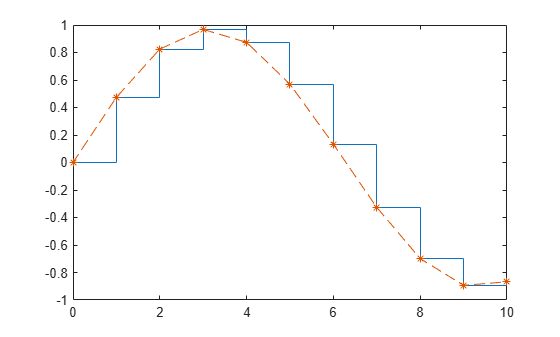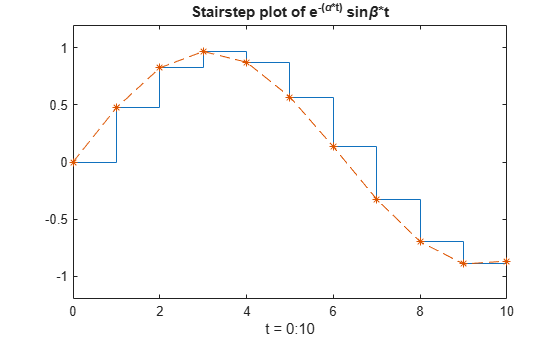# Overlay Stairstep Plot and Line Plot

This example shows how to overlay a line plot on a stairstep plot.

Define the data to plot.

alpha = 0.01; beta = 0.5; t = 0:10; f = exp(-alpha*t).*sin(beta*t);

Display f as a stairstep plot. Use the hold function to retain the stairstep plot. Add a line plot of f using a dashed line with star markers.

stairs(t,f) hold on plot(t,f,'--*') hold offUse the axis function to set the axis limits. Label the x-axis and add a title to the graph.

axis([0,10,-1.2,1.2]) xlabel('t = 0:10') title('Stairstep plot of e^{-(\alpha*t)} sin\beta*t')